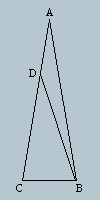All about flooble | fun stuff | Get a free chatterbox | Free JavaScript | Avatarsperplexus dot infoMeasure that angle III (Posted on 2004-09-07)Triangle ABC has side AB=AC, and angle BAC = 20 degrees.

D is a point on side AC with AD=BC. Find angle DBC.

Solve this without trigonometry.

 See The Solution Submitted by Brian Smith Rating: 3.2000 (5 votes)Comments: ( Back to comment list | You must be logged in to post comments.)Silly guess| Comment 5 of 25 |Picture the triangle differently, and see if we can extrapolate an answer.  Suppose angle A is not 20 degrees, but instead is 60 degrees.  In that case, ABC would be equilateral, and point D would coincide with point C.  Our answer for angle DBC would be zero degrees.

Now suppose instead, that angle A is very close to zero degrees.  Then side BC would be nearly zero and the angles at C and B would be nearly 90 degrees, as would be angle DBC.

If angle A were 60, then answer would be 0
If angle A were 0, then answer would be 90

So, if angle A is 20, is then answer we want:  60 degrees??
(can anyone prove this is a linear relationship?)

 Posted by Larry on 2004-09-08 07:45:17Please log in:

 Search: Search body:
Forums (1)